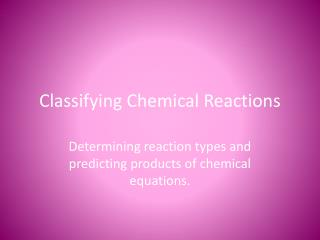DownloadDownload PresentationClassifying Chemical Reactions

# Classifying Chemical Reactions

Télécharger la présentation## Classifying Chemical Reactions

- - - - - - - - - - - - - - - - - - - - - - - - - - - E N D - - - - - - - - - - - - - - - - - - - - - - - - - - -
##### Presentation Transcript

1. Classifying Chemical Reactions Determining reaction types and predicting products of chemical equations.

2. Reaction Types • There is an unlimited number of reactions that may occur in the real world. • To make learning them easier, we classify them according to what is taking place. • Makes predicting products MUCH easier.

3. Combustion Reactions • Often used to generate energy. • Combustion Reaction – the oxidation of an organic compound, in which heat is released. • One reactant is a hydrocarbon and the other must be ______________. • Products are always carbon dioxide and water.

4. Combustion Reactions Examples: ___C3H8 + ___O2 ___H2O + ___CO2 ___C2H5OH + ___  ___ + ___ Write down the combustion of CH4

5. Synthesis Reaction • The word synthesis means “to put together” • Synthesis Reaction – a reaction in which two or more substances combine to form a new compound. • The reactants in many of these reactions are two elements or two small compounds.

6. Synthesis Reactions C + O2 CO22C + O2  2CO CaO(s) + H2O(l)  Ca(OH)2(s) Predict the products of the following synthesis rxns: K + Cl2  Mg + O2 

7. Decomposition Reaction • Decomposition reactions are the opposite of synthesis reactions – they have only one reactant. • In a decomposition reaction, a single compound breaks down, often with the input of energy, into two or more elements or simpler compounds.

8. Decomposition Reactions Water breaks down into its simpler components when introduced to electricity: 2H2O(l)---------> 2H2(g) + O2(g) Compounds made up of three or more elements usually do not decompose into those elements. CaCO3(s) -------> CaO(s) + CO2(g) Electricity Heat

9. Practice So Far: • Predict the product(s) and write a balanced equation for each of the following reactions: • The reaction of butane, C4H10, with oxygen. • The reaction of water and calcium oxide • The reaction of lithium with oxygen • The decomposition of carbonic acid.

10. Displacement Reactions • Single displacement (replacement) reaction- • A single element reacts with a compound and displaces another element of the same charge from the compound. • Examples: 2Al(s) + 3CuCl2(aq)  2AlCl3(aq) + 3Cu(s) • Predict the following products: CuSO4(aq) + Zn(s)

11. Activity Series, Page 281 • Used to make predictions about displacement reactions. • In the activity series, elements are arranged in order of activity with the most active on top. • In general, an element can displace those listed below it but not those above it. • You could also predict that no reaction will happen, such as when silver is put into a copper (II) nitrate soln.

12. Displacement Reactions • Double displacement (replacement) reaction- • Two compounds react with one another and swap cations / anions. • Examples: HCl(aq) + NaOH(aq) HOH(l) + NaCl(s) • Predict the following products: Cu(NO3)2(aq) + Al2(SO4)3(s)

13. Practice Classifying Reactions • Balance each of the equations below, and indicate the type of reaction for each equation ___Cl2(g) + ___NaBr(aq) ___NaCl(aq) + ___Br2(l) ___CaO(s) + ___H2O  ___Ca(OH)2(aq) ___Ca(ClO3)2(s)  ___CaCl2(s) + ___O2(g)

14. Practice Classifying Reactions • Balance each of the equations below, and indicate the type of reaction for each equation ___C8H18(l) + ___O2(g) ___CO2(g) + ___H2O(l) __Zn(s) + __CuBr2(aq)  __ZnBr2(aq) + __Cu(s) __AgNO3(aq) + __K2SO4(aq)  __Ag2SO4 + __KNO3(aq)

15. Practice With Activity Series • Predict whether a reaction would occur when the materials indicated are brought together. For each reaction that would occur, complete and balance the equation. ___Ag(s) + ___H2O(l) ___Mg(s) + ___Cu(NO3)2(aq) ___Al(s) + ___O2(g) ___H2SO4(aq) + ___KOH(aq)

16. Predicting Products • Predict the products, write a balanced equation, and identify the type of reaction for each of the following reactions. HgO C3H7OH + O2 Zn + CuSO4 

17. Predicting Products • Predict the products, write a balanced equation, and identify the type of reaction for each of the following reactions. BaCl2 + Na2SO4  Zn + F2  C5H10 + O2 

18. Finishing up the Chapter tomorrow (Net Ionic Equations). • Test Coming up!! • Start Practicing NOW! • Describing, Balancing, and Classifying Reactions